## Example Questions

### Example Question #1 : Expressions

Which of the following is equivalent to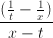? Assume that denominators are always nonzero.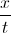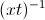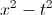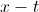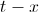Explanation:

We will need to simplify the expression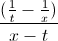. We can think of this as a large fraction with a numerator of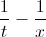and a denominator of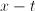.

In order to simplify the numerator, we will need to combine the two fractions. When adding or subtracting fractions, we must have a common denominator.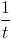has a denominator of, and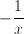has a denominator of. The least common denominator that these two fractions have in common is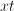. Thus, we are going to write equivalent fractions with denominators of.

In order to convert the fraction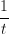to a denominator with, we will need to multiply the top and bottom by.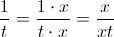Similarly, we will multiply the top and bottom ofby.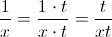We can now rewriteas follows:=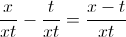Let's go back to the original fraction. We will now rewrite the numerator:=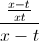To simplify this further, we can think ofas the same as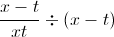. When we divide a fraction by another quantity, this is the same as multiplying the fraction by the reciprocal of that quantity. In other words,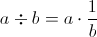.=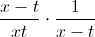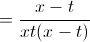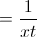Lastly, we will use the property of exponents which states that, in general,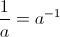.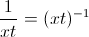The answer is.

### Example Question #1 : How To Divide Rational Expressions

Simplify: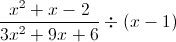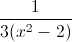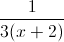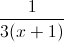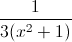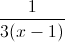Explanation:

Multiply by the reciprocal of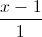.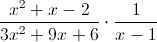Factor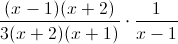Divide by common factors.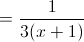### Example Question #1 : Expressions

Solve for: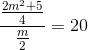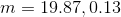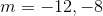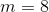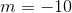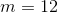Explanation:

To tackle this problem, you need to invert and multiply: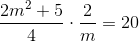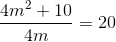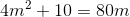Here we see that we have created a quadratic equation. Therefore, we get all terms to one side, set it equal to zero and use the quadratic formula to solve.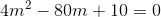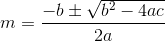where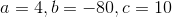Plugging these values in we get the following: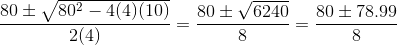### Example Question #2 : Expressions

Express the following as a single rational expression: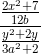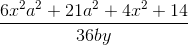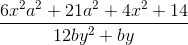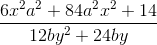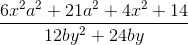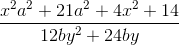Explanation:

To divide one rational expression by another, invert and multiply: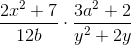Remember to foil the numerator meaning, multiply the first components of each binomial. Then multiply the outer components of each binomial. After that, multiply the inner components together, and lastly, multiply the components in the last position of the binomials together.

This arrives at the following: